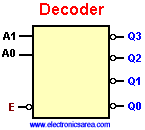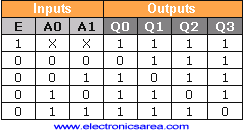# What is a Binary Decoder?

The Binary Decoder is a device that accepts a digital input (in binary form) and it activates one of its outputs. This device has several outputs, and the one that is activated is chosen by the code applied to the inputs. We can obtain 2n possible combinations with an n-bit code.

If you have a 3-bit code (3 inputs), it will be 8 (23) possible combinations. One particular code combination will only activate one output. For example: To activate the Q2 output we should place a binary number 2 (102)2 to 4 decoder

In a 2 to 4 decoder (2 inputs and 4 outputs), we can put one input binary code (00, 01, 10, 11) and it will activate only one of the four possible outputs. (Q0, Q1, Q2 Q3). Looking at the diagram above we can see that the E input is an active low input and all the Q are active low outputs

This means that when A0 = 0 and A1 = 0, we are choosing the Q0 output, and it (Q0) will be on “low” voltage level, while all other outputs (Q1, Q2 and Q3) will be on “high”voltage level.

In the same way, when the E input is on “low” level, the decoder is enabled. When the E input is on “high” level the decoder is disabled and no input on pins A0 and A1 will take effect. See the truth table.

## 2 to 4 Binary Decoder truth table2 to 4 Binary Decoder Truth Table

There are also 3 to 8 decoders (3 inputs and 8 outputs), 4 to 16 decoders (4 inputs and 16 outputs), and so on.

Notes:

• X means that the input can be anything (don’t care)
• 1 = H = High, 0 = L = Low
•
•
•
•
•
•
•
•
•
•
•
•
•
•
•
•
•
•